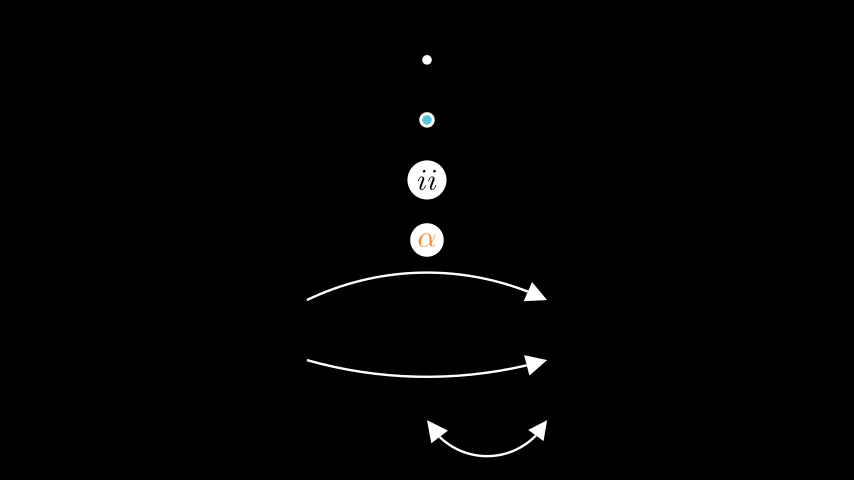# arc#

Mobjects that are curved.

Examples

Example: UsefulAnnotationsfrom manim import *

class UsefulAnnotations(Scene):
def construct(self):
m0 = Dot()
m1 = AnnotationDot()
m2 = LabeledDot("ii")
m3 = LabeledDot(MathTex(r"\alpha").set_color(ORANGE))
m4 = CurvedArrow(2*LEFT, 2*RIGHT, radius= -5)
m5 = CurvedArrow(2*LEFT, 2*RIGHT, radius= 8)
m6 = CurvedDoubleArrow(ORIGIN, 2*RIGHT)

self.add(m0, m1, m2, m3, m4, m5, m6)
for i, mobj in enumerate(self.mobjects):
mobj.shift(DOWN * (i-3))

class UsefulAnnotations(Scene):
def construct(self):
m0 = Dot()
m1 = AnnotationDot()
m2 = LabeledDot("ii")
m3 = LabeledDot(MathTex(r"\alpha").set_color(ORANGE))
m4 = CurvedArrow(2*LEFT, 2*RIGHT, radius= -5)
m5 = CurvedArrow(2*LEFT, 2*RIGHT, radius= 8)
m6 = CurvedDoubleArrow(ORIGIN, 2*RIGHT)

self.add(m0, m1, m2, m3, m4, m5, m6)
for i, mobj in enumerate(self.mobjects):
mobj.shift(DOWN * (i-3))



Classes

 AnnotationDot A dot with bigger radius and bold stroke to annotate scenes. AnnularSector A sector of an annulus. Annulus Region between two concentric Circles. Arc A circular arc. ArcBetweenPoints Inherits from Arc and additionally takes 2 points between which the arc is spanned. ArcPolygon A generalized polygon allowing for points to be connected with arcs. ArcPolygonFromArcs A generalized polygon allowing for points to be connected with arcs. Circle A circle. CubicBezier A cubic Bézier curve. CurvedArrow CurvedDoubleArrow Dot A circle with a very small radius. Ellipse A circular shape; oval, circle. LabeledDot A Dot containing a label in its center. Sector A sector of a circle. TipableVMobject Meant for shared functionality between Arc and Line.